Next: Poisson's Equation in Spherical Up: Potential Theory Previous: Spherical Harmonics

Laplace's Equation in Spherical Coordinates

Consider the general solution to Laplace's equation,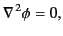(322)

in spherical coordinates. Let us write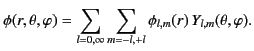(323)

It follows from Equation (308) that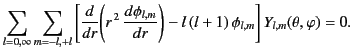(324)

However, given that the spherical harmonics are mutually orthogonal [in the sense that they satisfy Equation (311)], we can separately equate the coefficients of each in the above equation, to give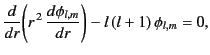(325)

for all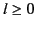and. It follows that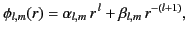(326)

where the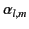and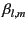are arbitrary constants. Hence, the general solution to Laplace's equation in spherical coordinates is written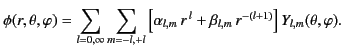(327)

If the domain of solution includes the origin then all of themust be zero, in order to ensure that the potential remains finite at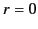. On the other hand, if the domain of solution extends to infinity then all of the(except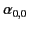) must be zero, otherwise the potential would be infinite at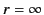.Next: Poisson's Equation in Spherical Up: Potential Theory Previous: Spherical Harmonics
Richard Fitzpatrick 2014-06-27